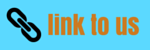# Law of Conservation of linear momentum

## (3) Law of Conservation of linear momentum

• Law of conservation of linear momentum is a extremely important consequence of Newton's third law of motion in combination with the second law of motion
• Consider two particles of mass m1 and m2 interacting with each other and forces acting on these particles are only the ones they exert on each other.
• Let F12 be the force exerted by the particle 2 on particle 1 having mass m1 and velocity v1 and F21=-F12 be the force exerted by the particle 1 on particle 2 having mass m2 and velocity v2
• Applying newton second law of each particle on each partcile

F12=m1(dv1/dt)
and F21=m2(dv2/dt)
• from newton's third law of motion
F21=-F12
or m1(dv1/dt) + m2(dv2/dt)=0
Since mass of the particles are not varying with time so we can write
(d/dt)(m1v1 +m2v2)=0
or m1v1 +m2v2=constant                 --(13)
• we have already defined the quantity mv as the momentum of the particle
• Thus we conclude that when two particles are subjected only to their mutual interactions ,the sum of the momentums of the bodies remains constant in time or we can say the total momentum of the two particles does not change becuase of the any mutual interactions between them
• For any kind of force between two particles then sum of the momentum ,both before and after the action of force should be equal i.e total momentum remains constant

• We thus arrive to the statment of principle of conservation of linear momentum
" when no resultant external force acts on system ,the total momentum of the system remains constant in magnitude and direction"
• In absence of external forces for a number of interacting particles,law of conservation of linear momentum can be expressed as
m1v1 +m2v2+m3v3+m4v4+...=constant
• Law of conservation of linear momentum is one of the most fundamental and important principle of mechanics

• This principle also holds true even if the forces between the interacting particles is not conservative

• Once again ,the total momentum of two or any number of particles of interacting particles is constant if they are isolated
from outside influences (or no resultant external forces is acting on the particles)

Watch this tutorial for more information on Position ,distance and displacement